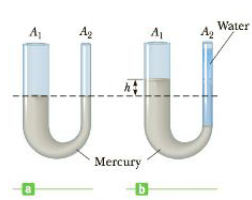# Mercury is poured into a U-tube as shown in Figure P9.10a. The left arm of the cube has cross-sectional area A 1 of 10.0 cm 2 , and the right arm has a cross-sectional area A 2 of 5.00 cm 2 . One hundred grams of water are then poured into the right arm as shown in Figure P9.10b. (a) Determine the length of the water column in the right arm of the U-tube. (b) Given that the density of mercury is 13.6 g/cm 3 , what distance h does the mercury rise in the left arm? Figure P9.10### College Physics

11th Edition
Raymond A. Serway + 1 other
Publisher: Cengage Learning
ISBN: 9781305952300### College Physics

11th Edition
Raymond A. Serway + 1 other
Publisher: Cengage Learning
ISBN: 9781305952300

#### Solutions

Chapter
Section
Chapter 9, Problem 10P
Textbook Problem

## Mercury is poured into a U-tube as shown in Figure P9.10a. The left arm of the cube has cross-sectional area A1 of 10.0 cm2, and the right arm has a cross-sectional area A2 of 5.00 cm2. One hundred grams of water are then poured into the right arm as shown in Figure P9.10b. (a) Determine the length of the water column in the right arm of the U-tube. (b) Given that the density of mercury is 13.6 g/cm3, what distance h does the mercury rise in the left arm?Figure P9.10

Expert Solution

(a)

To determine
The length of the water column in the right arm of the U-tube.

### Explanation of Solution

The volume of the water that poured into the right arm of the U-tube is given by Vw=mw/ρw and now the height at which the column has to be filled calculated as Vw=mw/ρw=A2hwhw=mw/(ρwA2) .

Given info: The right arm cross-sectional area of the U-tube is 5.00cm2 , mass of water is 100g , and the density of water is 1.00g/cm3 .

The formula for the length of the water column in the right arm of the U-tube is,

hw=mwρwA2

• mw is mass of the water
Expert Solution

(b)

To determine
The distance h rises in the left arm

### Want to see the full answer?

Check out a sample textbook solution.See solution

### Want to see this answer and more?

Bartleby provides explanations to thousands of textbook problems written by our experts, many with advanced degrees!

See solution